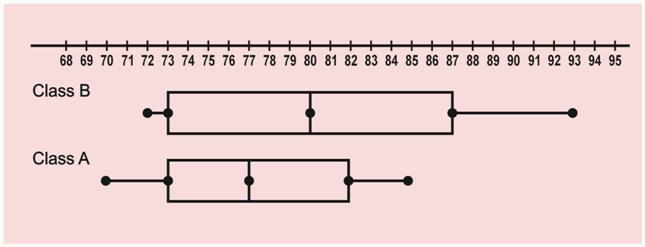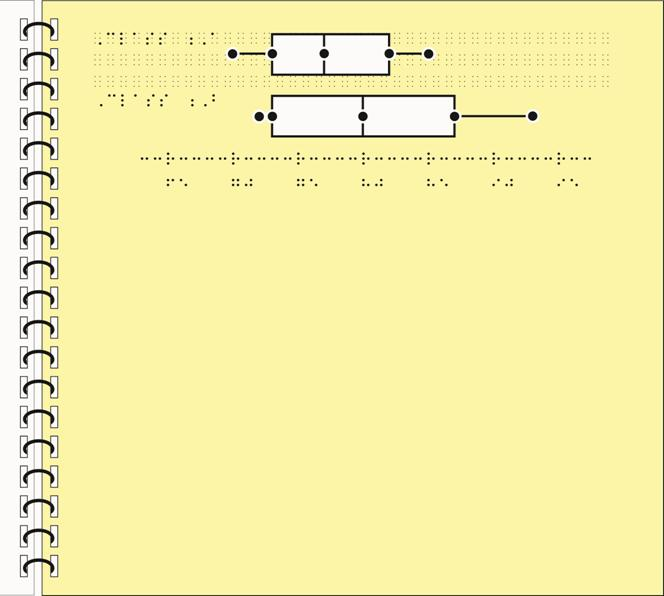### Box-and-Whisker Plot (Horizontal)

The following points indicate the braille code, format rules, and design techniques that were used for this tactile graphic example.

• Nemeth braille code
• intervals of 5 units only are labeled to allow more space for braille labeling (2.1)
• addition of value 65 on number line, because values are in intervals of 5; print number line starts at 68 (2.1)
• placement of box plot labels (Class A and Class B) at left margin (2.7)
• spacing of at least 1/8 inch (3 millimeters) between each component (number line, box plot, and labels) (2.11)
• use of Special Symbols page to explain braille dot symbols (5.13, 6.5.1.13)
• non-use of numeric indicators for values on number line (6.5.1.1)
• non-use of arrowheads on number line in braille, follows print (6.5.1.3)
• alignment of first digit of numeric label with regular scale mark (6.5.1.8)
• combination of braille symbol representation for number line and collage or digital file to produce box plots (6.5.3.3)
• movement of box plots above number line; is a mirror image of the box plots below number line in print (6.5.3.6)
• size of plotted points is uniform and larger than a braille dot (6.5.3.7)
• placement of box line closest to number line, no more than 1/4 inch (6 millimeters) from component (6.5.3.11)

### Box-and-Whisker Plot (Horizontal)### Box-and-Whisker Plot (Horizontal)